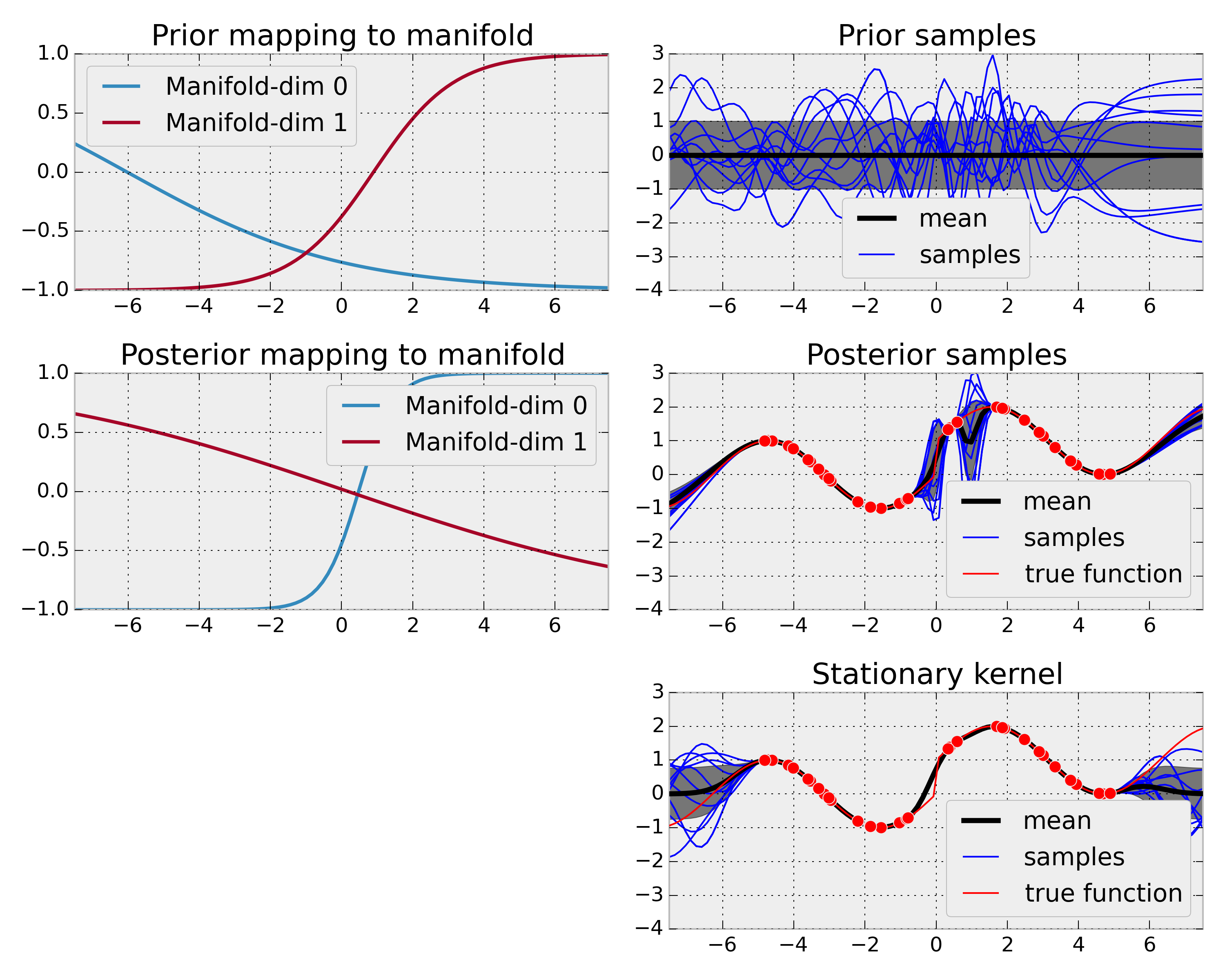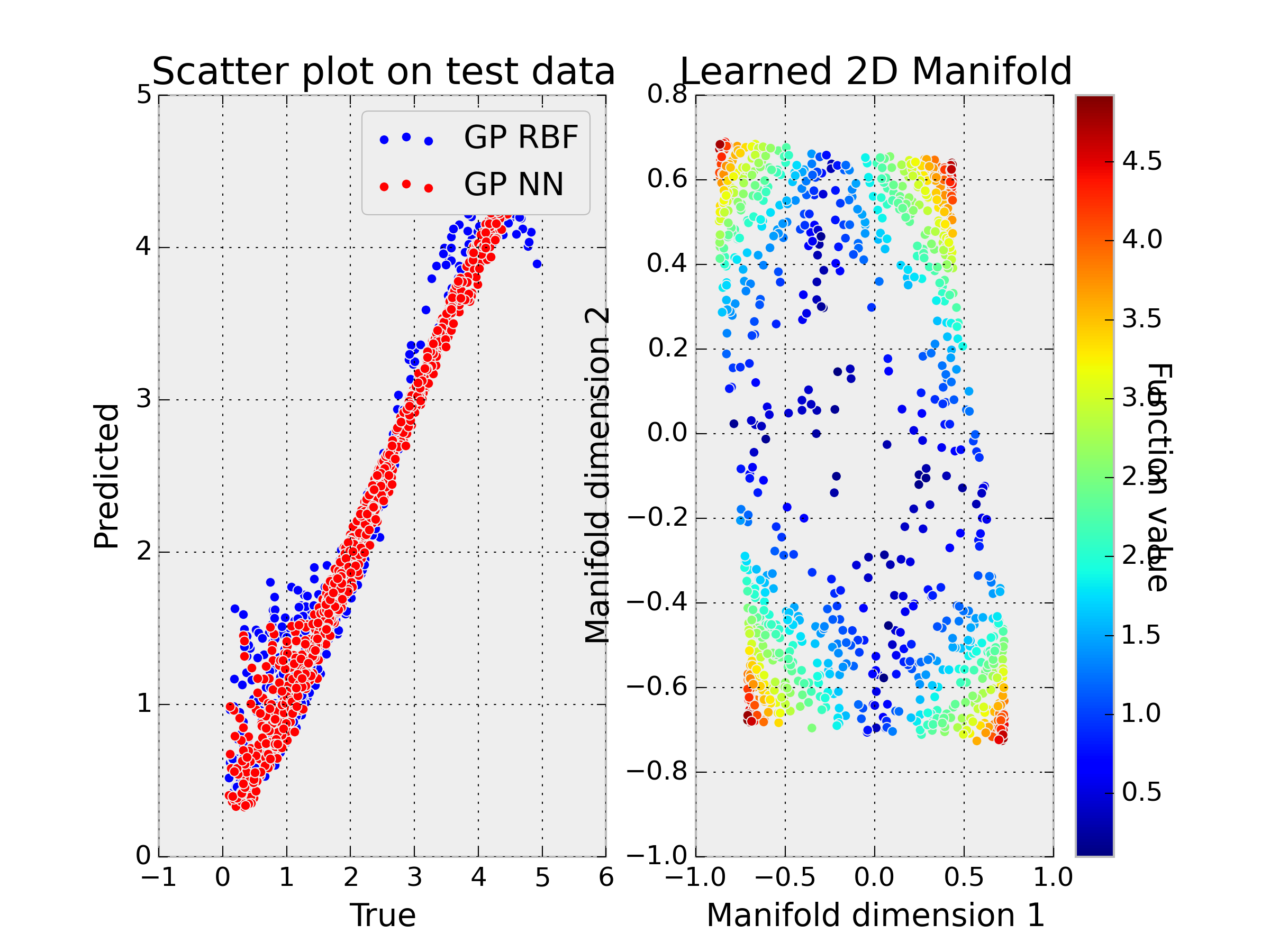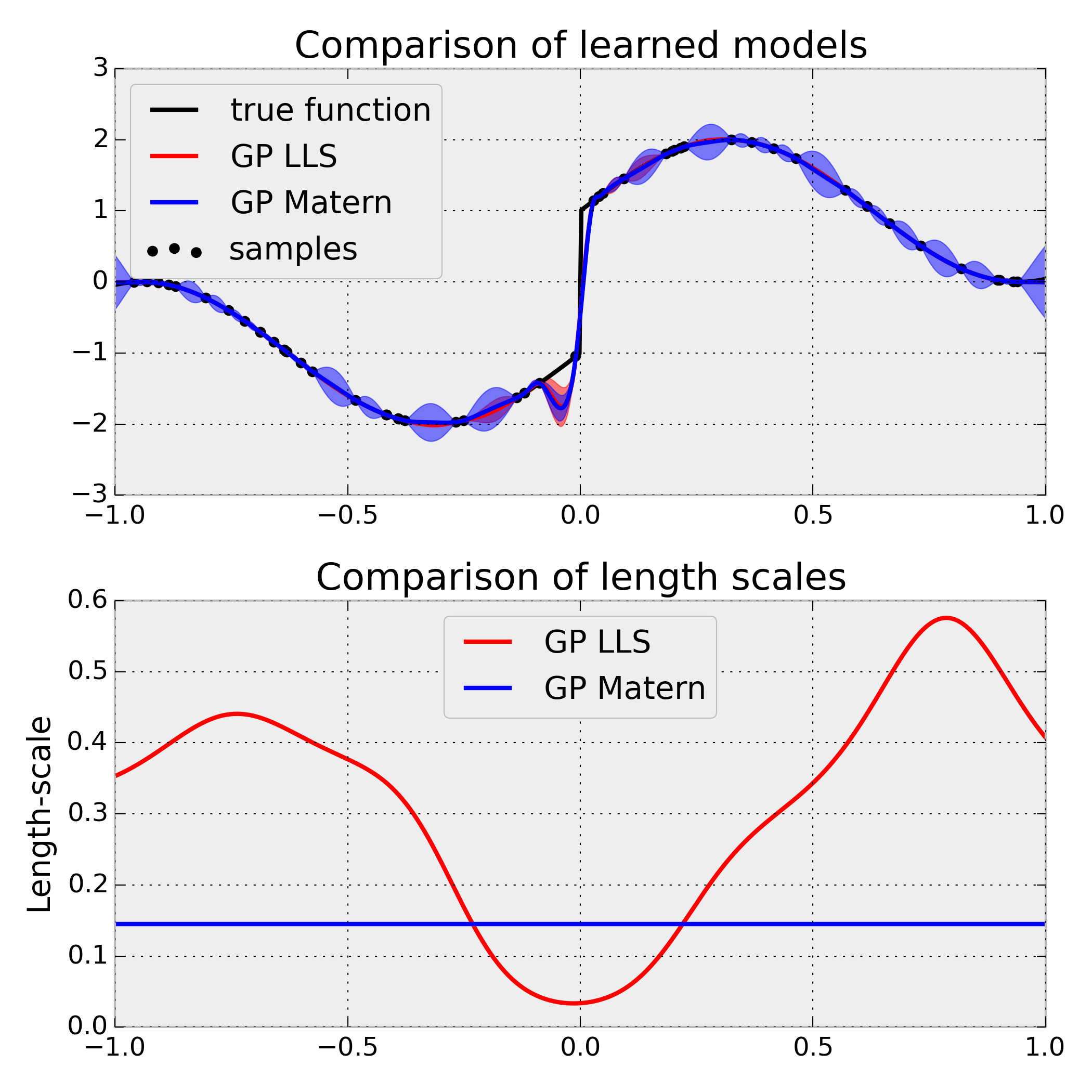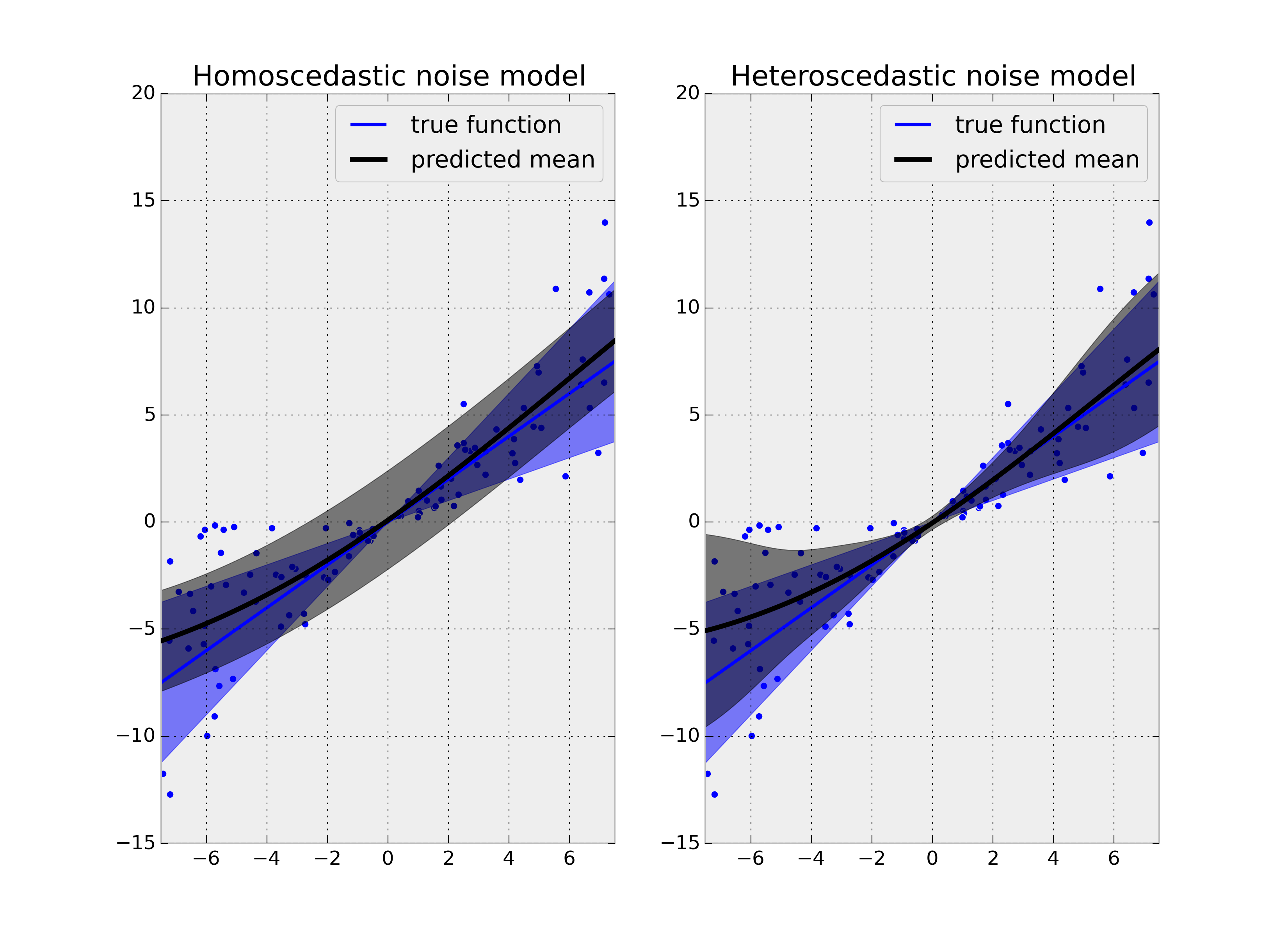# Additional Kernels for sklearn's new Gaussian Processes

Starting from version 0.18 (already available in the post-0.17 master branch), scikit-learn will ship a completely revised Gaussian process module, supporting among other things kernel engineering. While scikit-learn only ships the most common kernels, the gp_extra project contains some more advanced, non-standard kernels that can seamlessly be used with scikit-learn's GaussianProcessRegressor. The following kernels are included at the moment:

• ManifoldKernel: Non-stationary correlation model based on manifold learning. This non-stationary kernel consists internally of two parts: a mapping from the actual data space onto a manifold and a stationary kernel on this manifold. The mapping is realized by a neural network whose architecture can be specified externally. The parameters of this network are learned along with the length scales of the Gaussian process, typically such that the marginal likelihood or the posterior probability of the GP are maximized. Any common stationary kernel can then be used on top of this manifold.
• LocalLengthScalesKernel: Non-stationary kernel based on local smoothness estimates. This non-stationary correlation model learns internally point estimates of local smoothness using a second-level Gaussian Process. For this, it selects a subset of the training data and learns length-scales at this specific points. These length scales are generalized using the second-level Gaussian Process. Furthermore, global (isotropic or anisotropic) length scales are learned for both the top-level GP and the length-scale GP.
• HeteroscedasticKernel: Kernel which learns a heteroscedastic noise model. This kernel learns for a set of prototypes values from the data space explicit noise levels. These exemplary noise levels are then generalized to the entire data space by means for kernel regression.

## Examples¶

### Illustration how the ManifoldKernel can be used to deal with discontinuities¶

Source: plot_gpr_discontinuity.py

The ManifoldKernel allows to learn a mapping from low-dimensional input space (1d in this case) to a higher-dimensional manifold (2d in this case). Since this mapping is non-linear, this can be effectively used for turning a stationary base kernel into a non-stationary kernel, where the non-stationarity is learned. In this example, this used to learn a function which is sinusoidal but with a discontinuity at x=0. Using an adaptable non-stationary kernel allows to model uncertainty better and yields a better extrapolation beyond observed data in this example.### Illustration how ManifoldKernel can exploit data on lower-dimensional manifold¶

Source: plot_gpr_manifold.py

This example illustrates how the ManifoldKernel allows exploiting when the function to be learned has a lower effective input dimensionality (2d in the example) than the actual observed data (5d in the example). For this, a non-linear mapping (represented using an MLP) from data space onto manifold is learned. A stationary GP is used to learn the function on this manifold.

In the example, the ManifoldKernel is able to nearly perfectly recover the original square 2d structure of the function input space and correspondingly learns to model the target function better than a stationary, anisotropic GP in the 5d data space.### Illustration how the LocalLengthScalesKernel can with discontinuities¶

Source: plot_gpr_lls.py

The LocalLengthScalesKernel allows learning local length scales in the data space and thus can identify areas, in which broader and more narrow generalization is appropriate. This is illustrated on a simple sinusoidal function with a discontinuity at X=0. Because of this discontinuity, a stationary Matern kernel is forced to reduce the global length-scale considerably. A LocalLengthScalesKernel, on the other hand, needs to reduce only the length-scale close to the discontinuity, and achieves a considerably larger log-marginal-likelihood accordingly.

The example illustrates also how a custom optimizer based on differential evolution can be used for GP hyperparameter-tuning. This is required here because the log-marginal-likelihood for the LocalLengthScalesKernel is highly multi-modal, which is problematic for gradient-based methods like L-BFGS.### Illustration how HeteroscedasticKernel can learn a noise model¶

Source: gpr_heteroscedastic_noise.py

A heteroscedastic kernel allows adapting to situations where different regions in the data space exhibit different noise levels. For this, the kernel learns for a set of prototypes values from the data space explicit noise levels. These exemplary noise levels are then generalized to the entire data space by means for kernel regression.

In the shown example, a homoscedastic and heteroscedastic noise kernel are compared. The function to be learned is a simple linear relationship; however, the noise level grows quadratically with the input. Both kernels allow capturing the mean equally well; however, the heteroscedastic kernel can considerably better explain the observed data (according to the log-marginal likelihood LML) and provide better noise estimates.## Installation¶

You will need the new Gaussian process implementation from scikit-learn. For this, install the current development version of scikit-learn (or scikit-learn version 0.18 once this is available)

git clone git@github.com:scikit-learn/scikit-learn.git
cd sklearn
sudo python setup.py install



Now, you can install kernel_extras

git clone git@github.com:jmetzen/gp_extras.git
cd gp_extras/gp_extras
sudo python setup.py install

This post was written as an IPython notebook. You can download this notebook.